# Sphere Widget#

The sphere widget can be enabled and disabled by the `pyvista.Plotter.add_sphere_widget()` and `pyvista.Plotter.clear_sphere_widgets()` methods respectively. This is a very versatile widget as it can control vertex location that can be used to control or update the location of just about anything.

We don’t have any convenient helper methods that utilize this widget out of the box, but we have added a lot of ways to use this widget so that you can easily add several widgets to a scene.

Let’s look at a few use cases that all update a surface mesh.

```
```

## Example A#

Use a single sphere widget

```import numpy as np
import pyvista as pv

# Create a triangle surface
surf = pv.PolyData()
surf.points = np.array(
[
[-10, -10, -10],
[10, 10, -10],
[-10, 10, 0],
]
)
surf.faces = np.array([3, 0, 1, 2])

p = pv.Plotter()

def callback(point):
surf.points = point

p.show_grid()
p.show()
```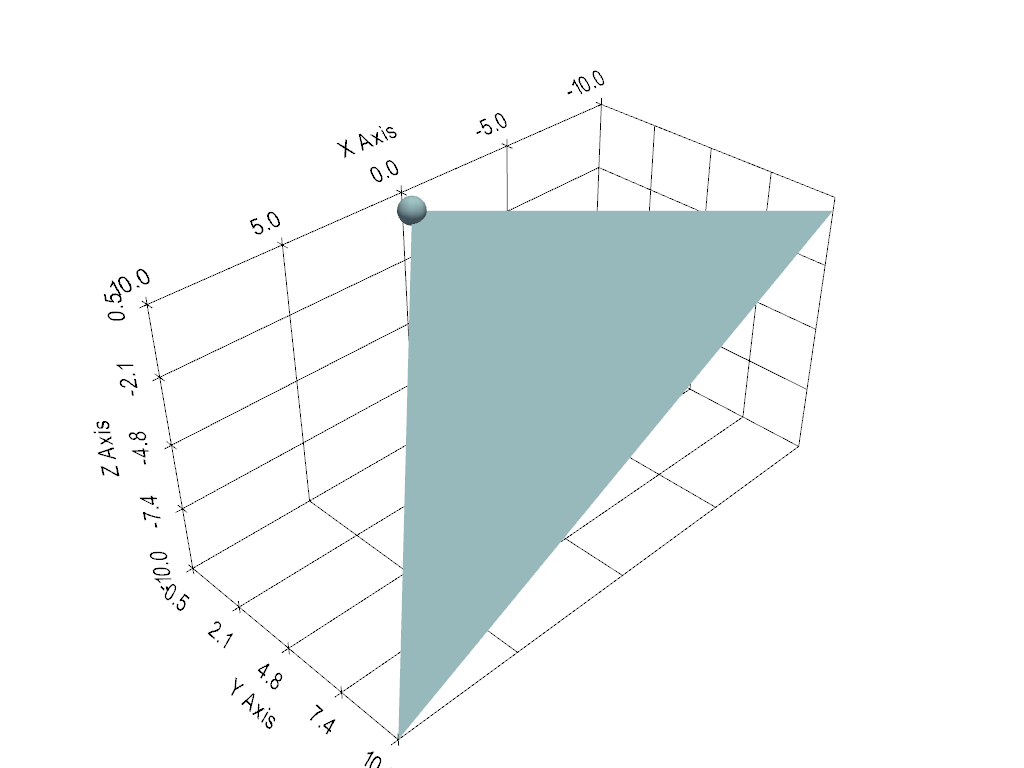And here is a screen capture of a user interacting with this## Example B#

Use several sphere widgets at once

```import numpy as np
import pyvista as pv

# Create a triangle surface
surf = pv.PolyData()
surf.points = np.array(
[
[-10, -10, -10],
[10, 10, -10],
[-10, 10, 0],
]
)
surf.faces = np.array([3, 0, 1, 2])

p = pv.Plotter()

def callback(point, i):
surf.points[i] = point

p.show_grid()
p.show()
```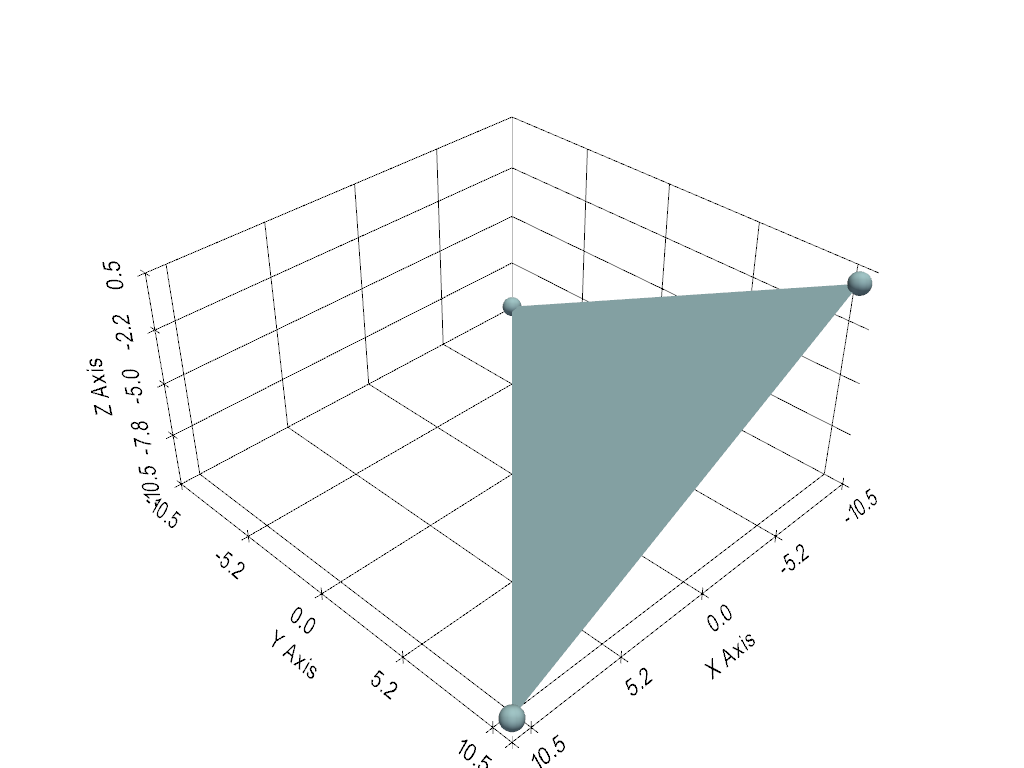And here is a screen capture of a user interacting with this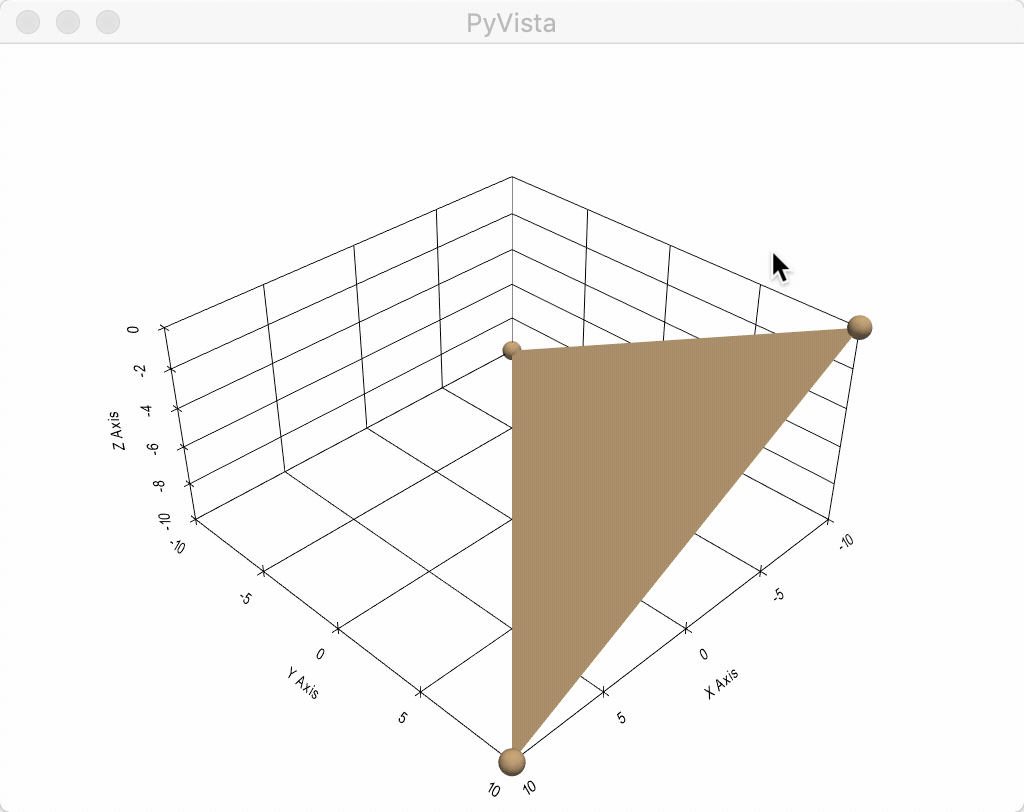## Example C#

This one is the coolest - use four sphere widgets to update perturbations on a surface and interpolate between them with some boundary conditions

```import numpy as np
import pyvista as pv
from scipy.interpolate import griddata

def get_colors(n):
"""A helper function to get n colors"""
from itertools import cycle

import matplotlib

cycler = matplotlib.rcParams['axes.prop_cycle']
colors = cycle(cycler)
colors = [next(colors)['color'] for i in range(n)]
return colors

# Create a grid to interpolate to
xmin, xmax, ymin, ymax = 0, 100, 0, 100
x = np.linspace(xmin, xmax, num=25)
y = np.linspace(ymin, ymax, num=25)
xx, yy, zz = np.meshgrid(x, y, )

# Make sure boundary conditions exist
boundaries = np.array([[xmin, ymin, 0], [xmin, ymax, 0], [xmax, ymin, 0], [xmax, ymax, 0]])

# Create the PyVista mesh to hold this grid
surf = pv.StructuredGrid(xx, yy, zz)

# Create some initial perturbations
# - this array will be updated inplace
points = np.array([[33, 25, 45], [70, 80, 13], [51, 57, 10], [25, 69, 20]])

# Create an interpolation function to update that surface mesh
def update_surface(point, i):
points[i] = point
tp = np.vstack((points, boundaries))
zz = griddata(tp[:, 0:2], tp[:, 2], (xx[:, :, 0], yy[:, :, 0]), method='cubic')
surf.points[:, -1] = zz.ravel(order='F')
return

# Get a list of unique colors for each widget
colors = get_colors(len(points))
```
```# Begin the plotting routine
p = pv.Plotter()

# Add the surface to the scene

# Add the widgets which will update the surface
p.show_grid()

# Show it!
p.show()
```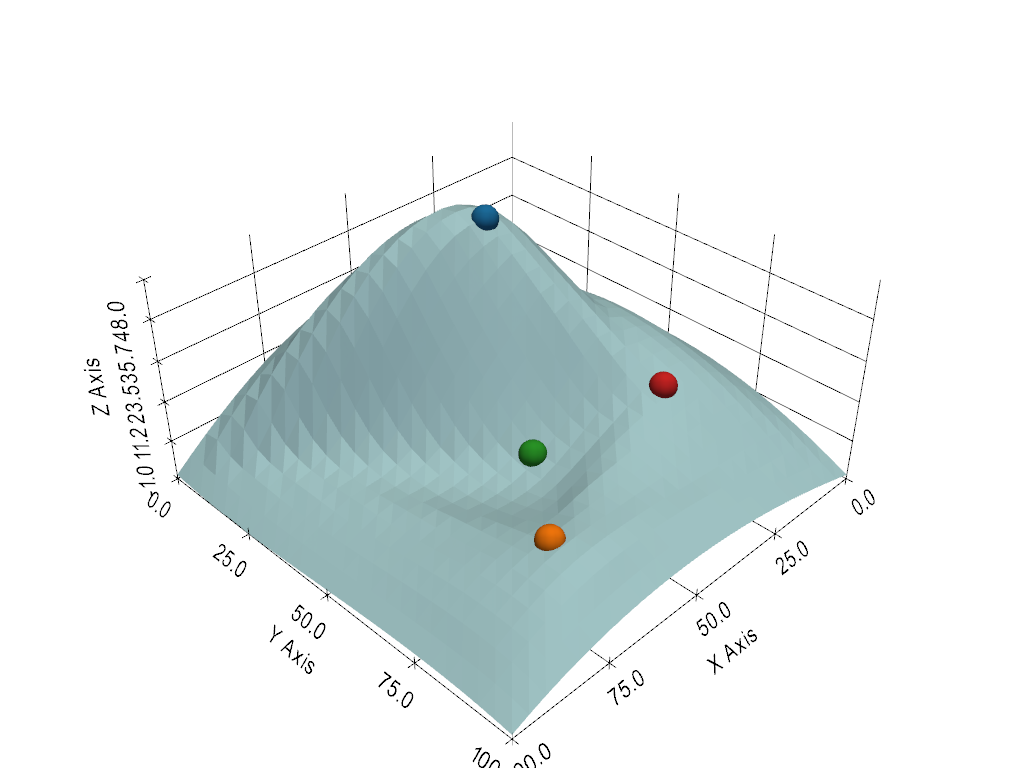And here is a screen capture of a user interacting with this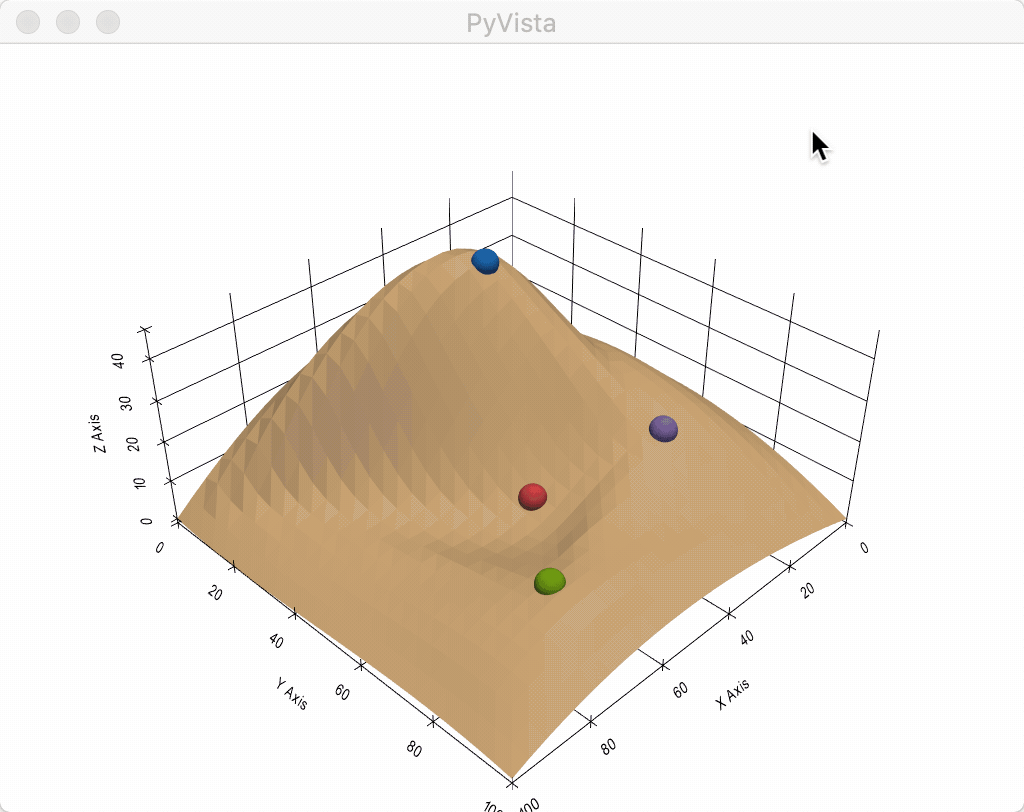Total running time of the script: ( 0 minutes 0.894 seconds)

Gallery generated by Sphinx-Gallery